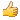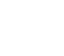# Thread: Converting between binary, decimal, and Oct

1. ## Converting between binary, decimal, and Oct

Aslaam alaykum, Does any 1 know how to convert between each 1??

I know how to convert binary to decimal and decimal to binary but how would you convert Octagnol to decimal.

And how would you convert Hex to decimal and vice versa.

And Hex to Octagnol

I need to learn how to convert from each of these, the only 1 I'm able to convert is binary to decimal and decimal to binary.

If any 1 knows I'd be grateful if you could explain really simplisticly, in the class room the teacher was going so fast and I asked him for help and he said just look on the board it's all on there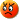2. ## Re: Converting between binary, decimal, and OctOriginally Posted by squiggle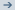Aslaam alaykum, Does any 1 know how to convert between each 1??

I know how to convert binary to decimal and decimal to binary but how would you convert Octagnol to decimal.
In the same way you convert binary to decimal, except your digits start from 0-7 and each postion from left to right is worth powers of 8.
example : octal(876)---> 8*(8^2)+7*(8^1)+6*(8^0)

And how would you convert Hex to decimal and vice versa.
Hex means base 16, binary is base 2, the same way you convert decimal to binary(keep dividing by 2) in this case you divide by 16

And Hex to Octagnol

I need to learn how to convert from each of these, the only 1 I'm able to convert is binary to decimal and decimal to binary.

If any 1 knows I'd be grateful if you could explain really simplisticly, in the class room the teacher was going so fast and I asked him for help and he said just look on the board it's all on thereIn short
In the decimal system each digit position is worth a power of 10 starting from (10^0) for the rightmost digit and increasing the power by one as you move to the left.
Here 10 is called the base.
example: when we write 876 we actually mean 800 + 70 + 6 i.e. 8*(10^2) + 7*(10^1) + 6*(10^0)

The decimal system is the way we humans have adjusted ourselves to communicate numerical information i.e. when i write 867 you automatically understand it to be equal to eight hundred and seventy six.

This can be extended to other systems like the binary system where the base is 2 and hence a number such as 1010 is worth : 1*(2^3)+0*(2^2)+1*(2^1) + 0*(2^0) i.e. 9.

hope this helps.3. ## Re: Converting between binary, decimal, and Oct

It helps alot brother thank you but I'm still confused, what would be the method to convert then.

like when you convert from decimal to binary you just do the following

Decimal 2

To convert it to binary I would just do this 128 64 32 16 8 4 2 1
`````````````````````````````````````` 0 0 0 0 0 0 1 0

and that gives me the number 2 in binary 00000010

isn't there an easy way to do it like that.

hmm for some reason in the example above I can't get the numbers in the correct place, but all I would do is right it out and then put 0s and 1s under each one. Like in the above example I would just put 1 under the number 2 and 0 under everything else and that will give me binary 2. Is there an easy way like this to do it.4. ## Re: Converting between binary, decimal, and Oct

^those links are very helpful brother Zak, I find it easy to learn like that with a step by step guide on how to do it.

Do you have any for Octagnol conversion?5. ## Re: Converting between binary, decimal, and Oct

hmm ok, I'm gonna have to pratice loads of these so it sticks in my head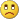6.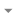Hide
Hey there!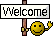Looks like you're enjoying the discussion, but you're not signed up for an account.

When you create an account, we remember exactly what you've read, so you always come right back where you left off. You also get notifications, here and via email, whenever new posts are made. And you can like posts and share your thoughts.